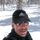cancel
Showing results for
Did you mean:New Member

## Removing Items from List if comparison criteria on next value(s) not met

I have a list of numbers and I need to ensure that each number is at least 2 * more than the next number and remove it if it's not.

For example, I have this list of numbers.  The orange highlighted numbers need to be removed from the list:This is what I've got so far, but I think the last step brings me right back to my original problem so I'm stuck again.

```#"List Unique Values" = List.Sort(List.Distinct(Table.Column(#"Table1","Value")), Order.Descending),
#"Table Unique Values" = Table.FromList(#"List Unique Values",Splitter.SplitByNothing(),{"Value"}),
#"Add Values List to Table" = Table.AddColumn(#"Table Unique Values", "Values", each #"List Unique Values"),
#"Expanded Values" = Table.ExpandListColumn(#"Add Values List to Table", "Values"),
#"Compare" = Table.AddColumn(#"Expanded Values","Compare", each if [Value] = [Values] then true else if [Value] > 2 * [Values] then true else false),```

Any thoughts?

Thanks!

1 ACCEPTED SOLUTIONNew Member

I was actually just looking at that link!  It ultimately helped me come up with the following solution:

```(values as list) as list =>
let
sorted = List.Sort(List.Distinct(values), Order.Descending),

comparingValues = (n as number, valuesToCompare as list) =>
let
valueToCompare = valuesToCompare{n},
Nullify = List.Transform(List.Sort(List.Distinct(valuesToCompare), Order.Descending),
each
if _ = null then null
else if _ = valueToCompare then _
else if _ > 2 * valueToCompare then _
else if _ < valueToCompare/2 then _
else null
),
RemoveNulls = List.RemoveItems(Nullify, {null})
in
RemoveNulls,

finalValues = List.Generate(
() => [n=0, myList = sorted, count=List.Count(sorted)],
each [n] <= [count],
each [n=[n]+1, myList=comparingValues([n], [myList]), count=List.Count([myList])]
)
in
finalValues```

I orginally tried not having the count variable in the List.Generate function but it kept throwing a "not enough elements in the enumeration" error.

Works great!

5 REPLIES 5Super User

Hi @estuelke ,

Not sure of your logic, but this is what your sentence says to me:

"I have a list of numbers and I need to ensure that each number is at least 2 * more than the next number and remove it if it's not."

So we start with the first number and compare it to the next number. Then the first number must be more than 2* greater than the next number then remove " it". What is "it"? the first number or the second?

49.97 *2 =    99.94 right? So remove the rest of the list? Because each number that is removed, then the first number that is left is then the next number to compare.

Nathaniel

Proud to be a Super User!New Member

Hi @Nathaniel_C ,

I want to compare the first number to the second number.  If the first is not 2 * greater than the second, remove the second and keep comparing down the list removing numbers until I find a number where the first is 2 * greater.

Then I want to traverse the newly updated list again, but starting with the second number, and so on...

So the end result would be a list where each number is at least 2 * greater than the one that follows.Super User

Hi @estuelke ,

Wow! Thanks for this opportunity! As you described the issue, my background in programming said that sounds like a loop.  Don't think so...however this blog post describes how to create a loop in M language. https://potyarkin.ml/posts/2017/loops-in-power-query-m-language/

Do you have programming background? Try it and let me know. Otherwise if I have some time, I will try it this weekend.

If this solves your issues, please mark it as the solution, so that others can find it easily. Kudos are nice too.
Nathaniel

Proud to be a Super User!New Member

I was actually just looking at that link!  It ultimately helped me come up with the following solution:

```(values as list) as list =>
let
sorted = List.Sort(List.Distinct(values), Order.Descending),

comparingValues = (n as number, valuesToCompare as list) =>
let
valueToCompare = valuesToCompare{n},
Nullify = List.Transform(List.Sort(List.Distinct(valuesToCompare), Order.Descending),
each
if _ = null then null
else if _ = valueToCompare then _
else if _ > 2 * valueToCompare then _
else if _ < valueToCompare/2 then _
else null
),
RemoveNulls = List.RemoveItems(Nullify, {null})
in
RemoveNulls,

finalValues = List.Generate(
() => [n=0, myList = sorted, count=List.Count(sorted)],
each [n] <= [count],
each [n=[n]+1, myList=comparingValues([n], [myList]), count=List.Count([myList])]
)
in
finalValues```

I orginally tried not having the count variable in the List.Generate function but it kept throwing a "not enough elements in the enumeration" error.

Works great!Super User

Sweet! It is amazing how much info is out there!

Nathaniel

Proud to be a Super User!